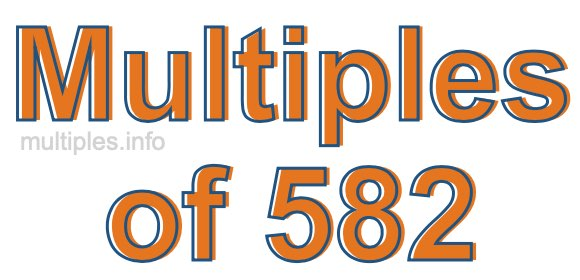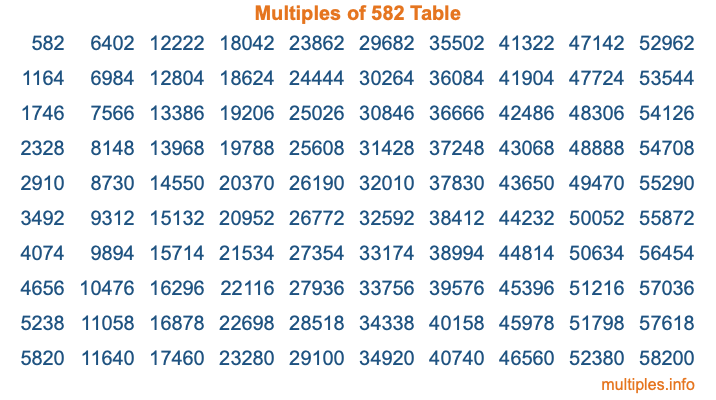Multiples of 582Welcome to the Multiples of 582 page. Here we will first teach you everything you will ever need to know about the multiples of 582, and then give you a study guide summary of everything we taught you to make sure you remember it all. Use this page to look up facts and learn information about the multiples of 582. This page will make you a multiples of five hundred eighty-two expert!

Definition of Multiples of 582
Multiples of 582 are all the numbers that when divided by 582 equal an integer. Each of the multiples of 582 are called a multiple. A multiple of 582 is created by multiplying 582 by an integer.

Therefore, to create a list of multiples of 582, you start with 1 multiplied by 582, then 2 multiplied by 582, then 3 multiplied by 582, and so on for as long as you want. Thus, the list of the first five multiples of 582 is 582, 1164, 1746, 2328, and 2910. To see a larger list of multiples of 582, see the printable image of Multiples of 582 further down on this page. We also have a category where you can choose any nth multiple of 582.

Multiples of 582 Checker
The Multiples of 582 Checker below checks to see if any number of your choice is a multiple of 582. In other words, it checks to see if there is any number (integer) that when multiplied by 582 will equal your number. To do that, we divide your number by 582. If the the quotient is an integer, then your number is a multiple of 582.

Is  a multiple of 582?

Least Common Multiple of 582 and ...
A Least Common Multiple (LCM) is the lowest multiple that two or more numbers have in common. This is also called the smallest common multiple or lowest common multiple and is useful to know when you are adding our subtracting fractions. Enter one or more numbers below (582 is already entered) to find the LCM.

Check out our LCM Calculator if you need more details about the Least Common Multiple or if you need the LCM for different numbers for adding and subtraction fractions.

nth Multiple of 582
As we stated above, 582 is the first multiple of 582, 1164 is the second multiple of 582, 1746 is the third multiple of 582, and so on. Enter a number below to find the nth multiple of 582.

th multiple of 582

Multiples of 582 vs Factors of 582
582 is a multiple of 582 and a factor of 582, but that is where the similarities end. All postive multiples of 582 are 582 or greater than 582. All positive factors of 582 are 582 or less than 582.

Below is the beginning list of multiples of 582 and the factors of 582 so you can compare:

Multiples of 582: 582, 1164, 1746, 2328, 2910, etc.

Factors of 582: 1, 2, 3, 6, 97, 194, 291, 582

As you can see, the multiples of 582 are all the numbers that you can divide by 582 to get a whole number. The factors of 582, on the other hand, are all the whole numbers that you can multiply by another whole number to get 582.

It's also interesting to note that if a number (x) is a factor of 582, then 582 will also be a multiple of that number (x).

Multiples of 582 vs Divisors of 582
The divisors of 582 are all the integers that 582 can be divided by evenly. Below is a list of the divisors of 582.

Divisors of 582: 1, 2, 3, 6, 97, 194, 291, 582

The interesting thing to note here is that if you take any multiple of 582 and divide it by a divisor of 582, you will see that the quotient is an integer.

Multiples of 582 Table
Below is an image of the first 100 multiples of 582 in a table. The table is in chronological order, column by column. The first column has the first ten multiples of 582, the second column has the next ten multiples of 582, and so on.The Multiples of 582 Table is also referred to as the 582 Times Table or Times Table of 582. You are welcome to print out our table for your studies.

Negative Multiples of 582
Although not often discussed or needed in math, it is worth mentioning that you can make a list of negative multiples of 582 by multiplying 582 by -1, then by -2, then by -3, and so on, to get the following list of negative multiples of 582:

-582, -1164, -1746, -2328, -2910, etc.

Multiples of 582 Summary
Below is a summary of important Multiples of 582 facts that we have discussed on this page. To retain the knowledge on this page, we recommend that you read through the summary and explain to yourself or a study partner why they hold true.

There are an infinite number of multiples of 582.

A multiple of 582 divided by 582 will equal a whole number.

582 divided by a factor of 582 equals a divisor of 582.

The nth multiple of 582 is n times 582.

The largest factor of 582 is equal to the first positive multiple of 582.

582 is a multiple of every factor of 582.

582 is a multiple of 582.

A multiple of 582 divided by a divisor of 582 equals an integer.

582 divided by a divisor of 582 equals a factor of 582.

Any integer times 582 will equal a multiple of 582.

Multiples of a Number
Here you can get the multiples of another number, all with the same attention to detail as we did for multiples of 582 on this page.

Multiples of
Multiples of 583
Did you find our page about multiples of five hundred eighty-two educational? Do you want more knowledge? Check out the multiples of the next number on our list!